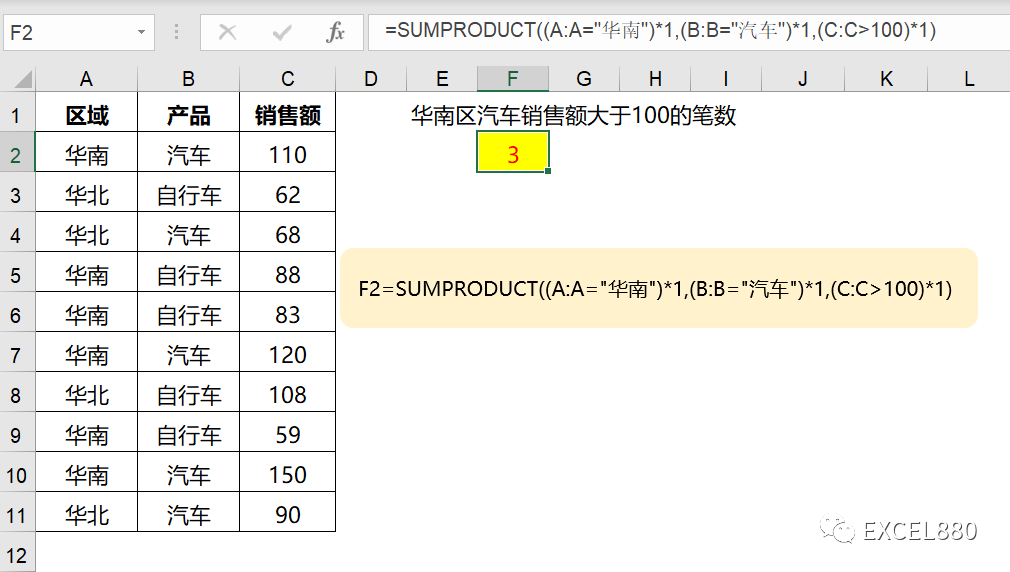# 过度吹捧的SUMPRODUCT函数 原来如此简单

86 次浏览

ALT+=一秒求和，CTRL+E 自动填充，类似的技能俨然成了流量密码，个个主播都在不停的讲，函数方面典型的就是SUMPRODUCT.

SUMPRODCT主要被吹嘘用于（多）条件求和，（多）条件计数，以下图所示的多条件计数为例，求华南区汽车销售额大于100的笔数。

=SUMPRODUCT((A:A=”华南”)*(B:B=”汽车”)*(C:C>100))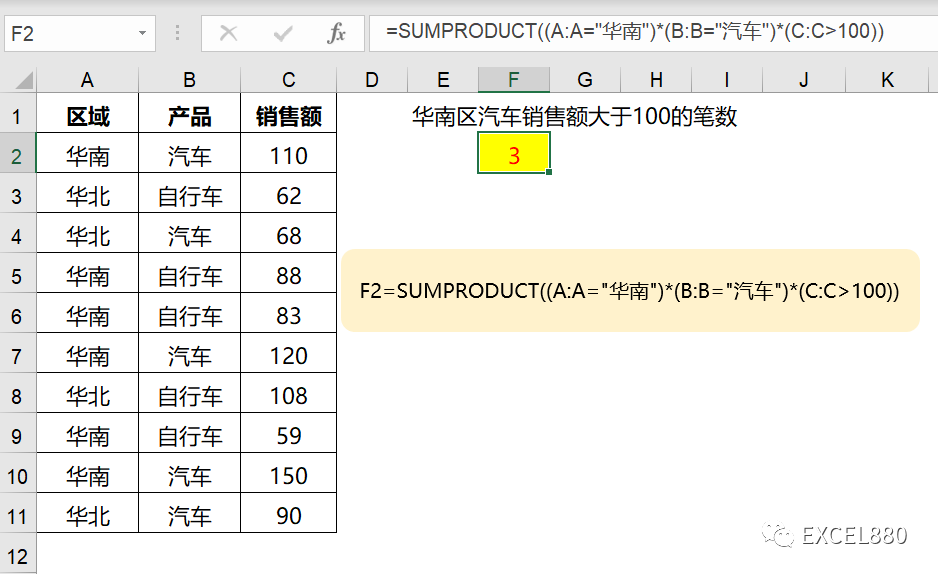=SUM((A:A=”华南”)*(B:B=”汽车”)*(C:C>100))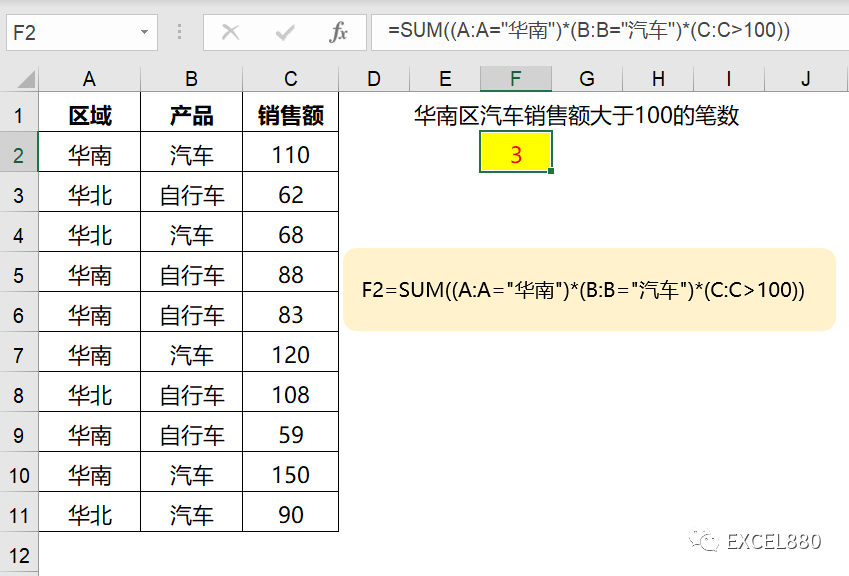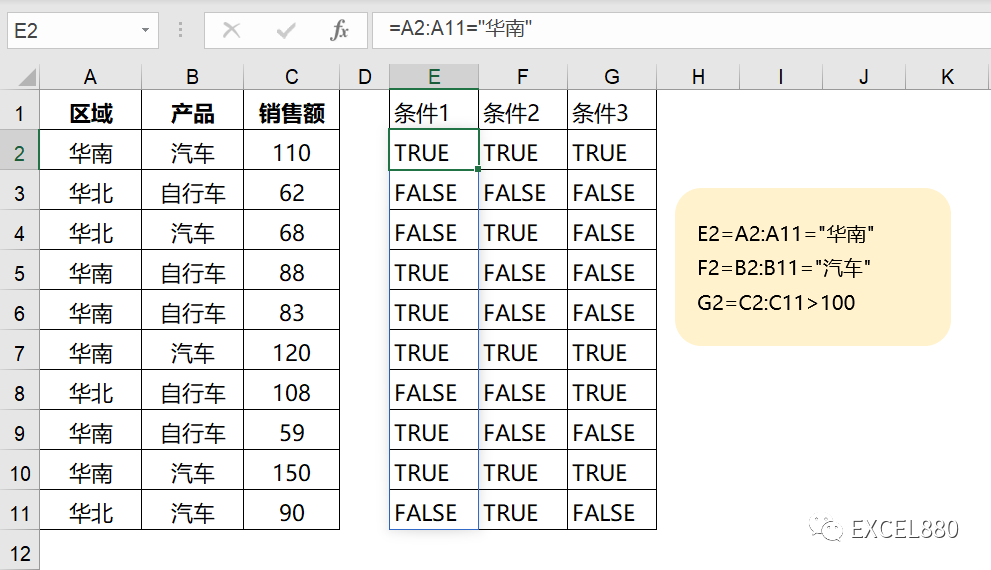=(A2:A11=”华南”)*(B2:B11=”汽车”)*(C2:C11>100)

Excel笔记：逻辑值TRUE和FALSE通过数学运算可转换为数字，TRUE=1,FALSE=0.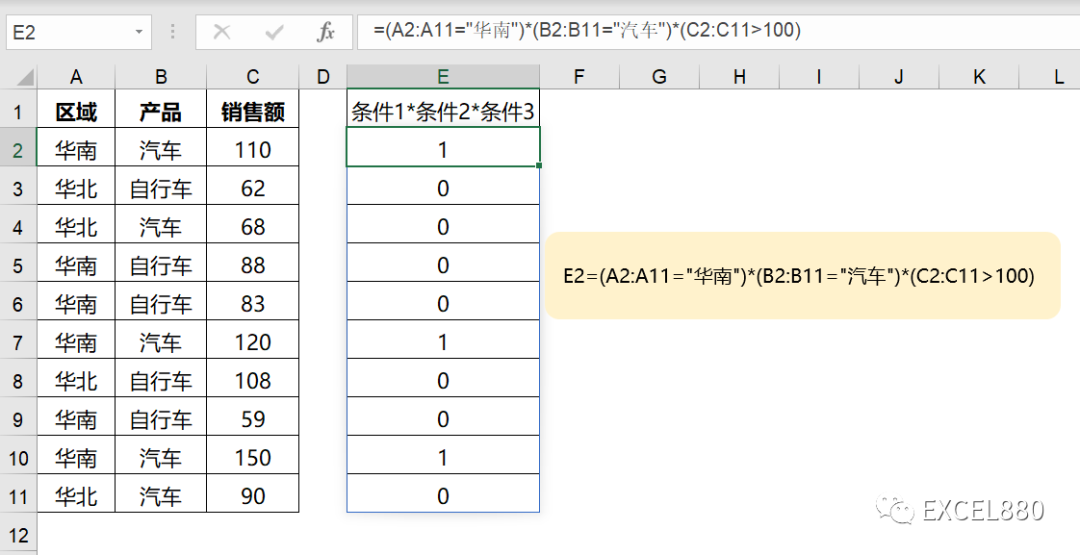=SUMPRODUCT((A:A=”华南”)*1,(B:B=”汽车”)*1,(C:C>100)*1)